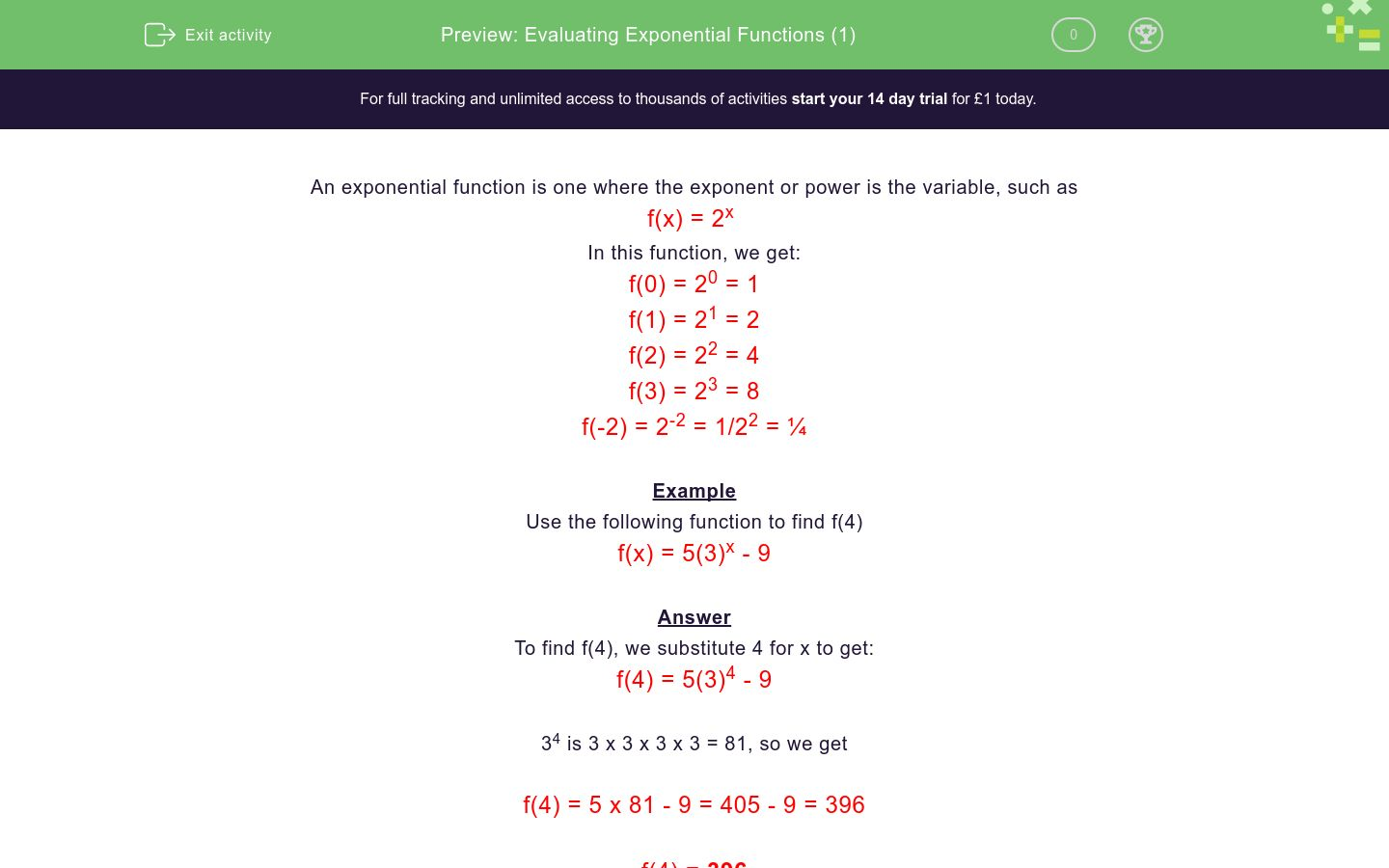# Evaluating Exponential Functions (1)

In this worksheet, students substitute numerical values into exponential functions.Key stage:  KS 4

Curriculum topic:  Algebra

Difficulty level:### QUESTION 1 of 10

An exponential function is one where the exponent or power is the variable, such as

f(x) = 2x

In this function, we get:

f(0) = 20 = 1

f(1) = 21 = 2

f(2) = 22 = 4

f(3) = 23 = 8

f(-2) = 2-2 = 1/22 = ¼

Example

Use the following function to find f(4)

f(x) = 5(3)x - 9

To find f(4), we substitute 4 for x to get:

f(4) = 5(3)4 - 9

34 is 3 x 3 x 3 x 3 = 81, so we get

f(4) = 5 x 81 - 9 = 405 - 9 = 396

f(4) = 396

Use the following function to find f(2)

f(x) = 3x

Use the following function to find f(4)

f(x) = 2x + 3

Use the following function to find f(2)

f(x) = 5(3)x - 7

Use the following function to find f(2)

f(x) = 2(3)x + 7

Use the following function to find f(4)

f(x) = 5x + 1

Use the following function to find f(0)

f(x) = 8x + 49

Use the following function to find f(0)

f(x) = 7(7)x + 4

Use the following function to find f(2)

f(x) = 12x - 44

Use the following function to find f(3)

f(x) = 7x - 44

Use the following function to find f(5)

f(x) = 3(4)x - 72

• Question 1

Use the following function to find f(2)

f(x) = 3x

9
EDDIE SAYS
32
• Question 2

Use the following function to find f(4)

f(x) = 2x + 3

19
EDDIE SAYS
24 + 3 = 16 + 3
• Question 3

Use the following function to find f(2)

f(x) = 5(3)x - 7

38
EDDIE SAYS
5 × 32 - 7 = 5 × 9 - 7
• Question 4

Use the following function to find f(2)

f(x) = 2(3)x + 7

25
EDDIE SAYS
2 × 32 + 7 = 2 × 9 + 7
• Question 5

Use the following function to find f(4)

f(x) = 5x + 1

626
EDDIE SAYS
54 + 1 = 625 + 1
• Question 6

Use the following function to find f(0)

f(x) = 8x + 49

50
EDDIE SAYS
80 + 49 = 1 + 49
• Question 7

Use the following function to find f(0)

f(x) = 7(7)x + 4

11
EDDIE SAYS
7 × 70 + 4 = 7 × 1 + 4
• Question 8

Use the following function to find f(2)

f(x) = 12x - 44

100
EDDIE SAYS
122 - 44 = 144 - 44
• Question 9

Use the following function to find f(3)

f(x) = 7x - 44

299
EDDIE SAYS
73 - 44 = 343 - 44
• Question 10

Use the following function to find f(5)

f(x) = 3(4)x - 72

3000
EDDIE SAYS
3 × 45 - 72 = 3 × 1024 - 72
---- OR ----

Sign up for a £1 trial so you can track and measure your child's progress on this activity.

### What is EdPlace?

We're your National Curriculum aligned online education content provider helping each child succeed in English, maths and science from year 1 to GCSE. With an EdPlace account you’ll be able to track and measure progress, helping each child achieve their best. We build confidence and attainment by personalising each child’s learning at a level that suits them.

Start your £1 trial